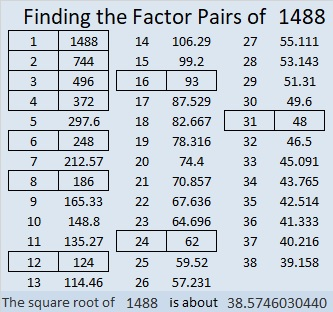# 1488 Chalk Art

Contents

### Geometric Chalk Art:

My grandchildren and their mom laid down some masking tape on a sidewalk panel to create lots of polygons. Then they colored it in beautifully with chalk before they removed most of the masking tape. My sidewalk never looked so good!Perhaps you would enjoy creating some mathematical chalk art on your sidewalk, too!

### Factors of 1488:

• 1488 is a composite number.
• Prime factorization: 1488 = 2 × 2 × 2 × 2 × 3 × 31, which can be written 1488 = 2⁴ × 3 × 31
• 1488 has at least one exponent greater than 1 in its prime factorization so √1488 can be simplified. Taking the factor pair from the factor pair table below with the largest square number factor, we get √1488 = (√16)(√93) = 4√93. The exponents in the prime factorization are 4, 1, and 1. Adding one to each exponent and multiplying we get (4 + 1)(1 + 1)(1 + 1) = 5 × 2 × 2 = 20. Therefore 1488 has exactly 20 factors.
• The factors of 1488 are outlined with their factor pair partners in the graphic below.### More about the Number 1488:

1488 is the difference of two squares in six different ways:
373² – 371² = 1488
188² – 184² = 1488
127² – 121² = 1488
97² – 89² = 1488
68² – 56² = 1488
43² – 19² = 1488

This site uses Akismet to reduce spam. Learn how your comment data is processed.